Grouping Of Images Quiz II, Non Verbal Reasoning

# Grouping Of Images Quiz II, Non Verbal Reasoning - Mechanical Engineering

Test Description

## 10 Questions MCQ Test General Aptitude for GATE - Grouping Of Images Quiz II, Non Verbal Reasoning

Grouping Of Images Quiz II, Non Verbal Reasoning for Mechanical Engineering 2023 is part of General Aptitude for GATE preparation. The Grouping Of Images Quiz II, Non Verbal Reasoning questions and answers have been prepared according to the Mechanical Engineering exam syllabus.The Grouping Of Images Quiz II, Non Verbal Reasoning MCQs are made for Mechanical Engineering 2023 Exam. Find important definitions, questions, notes, meanings, examples, exercises, MCQs and online tests for Grouping Of Images Quiz II, Non Verbal Reasoning below.
Solutions of Grouping Of Images Quiz II, Non Verbal Reasoning questions in English are available as part of our General Aptitude for GATE for Mechanical Engineering & Grouping Of Images Quiz II, Non Verbal Reasoning solutions in Hindi for General Aptitude for GATE course. Download more important topics, notes, lectures and mock test series for Mechanical Engineering Exam by signing up for free. Attempt Grouping Of Images Quiz II, Non Verbal Reasoning | 10 questions in 20 minutes | Mock test for Mechanical Engineering preparation | Free important questions MCQ to study General Aptitude for GATE for Mechanical Engineering Exam | Download free PDF with solutions
 1 Crore+ students have signed up on EduRev. Have you?
Grouping Of Images Quiz II, Non Verbal Reasoning - Question 1

### Directions to Solve In each of the following questions, group the given figures into three classes using each figure only once. Question - Group the given figures into three classes using each figure only once.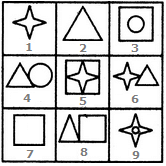Detailed Solution for Grouping Of Images Quiz II, Non Verbal Reasoning - Question 1

1, 2, 7 are simple geometrical figures.

3, 5, 9 have one element placed inside a different element.

4, 6, 8 have two different elements attached to each other.

Grouping Of Images Quiz II, Non Verbal Reasoning - Question 2

### Directions to Solve In each of the following questions, group the given figures into three classes using each figure only once. Question - Group the given figures into three classes using each figure only once.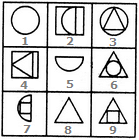Detailed Solution for Grouping Of Images Quiz II, Non Verbal Reasoning - Question 2

1, 3, 6 contain one complete circle each.

2, 5, 7 contain a semi-circle each.

4, 8, 9 contain a triangle each.

Grouping Of Images Quiz II, Non Verbal Reasoning - Question 3

### Directions to Solve In each of the following questions, group the given figures into three classes using each figure only once. Question - Group the given figures into three classes using each figure only once.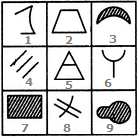Detailed Solution for Grouping Of Images Quiz II, Non Verbal Reasoning - Question 3

1, 6, 8 are figures composed of straight as well as curved lines.

3, 7, 9 are closed figures shaded by oblique line segments.

2, 4, 5 are figures composed of straight lines only.

Grouping Of Images Quiz II, Non Verbal Reasoning - Question 4
Directions to Solve

In each of the following questions, group the given figures into three classes using each figure only once.

Question -

Group the given figures into three classes using each figure only once.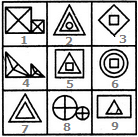Detailed Solution for Grouping Of Images Quiz II, Non Verbal Reasoning - Question 4

1, 4, 8 contain similar elements (not equal in size) each divided into four parts and attached to each other.

2, 5, 6 contain three elements (two of which are similar) placed one inside the other.

3, 7, 9 contain one element inside the other, which may or may not be similar.

Grouping Of Images Quiz II, Non Verbal Reasoning - Question 5
Directions to Solve

In each of the following questions, group the given figures into three classes using each figure only once.

Question -

Group the given figures into three classes using each figure only once.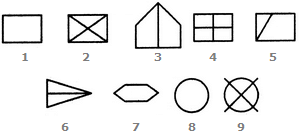Detailed Solution for Grouping Of Images Quiz II, Non Verbal Reasoning - Question 5

1, 7, 8 are all undivided geometrical figures.

3, 5, 6 are geometrical figures divided into two parts.

2, 4, 9 are geometrical figures divided into four parts.

Grouping Of Images Quiz II, Non Verbal Reasoning - Question 6
Directions to Solve

In each of the following questions, group the given figures into three classes using each figure only once.

Question -

Group the given figures into three classes using each figure only once.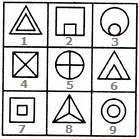Detailed Solution for Grouping Of Images Quiz II, Non Verbal Reasoning - Question 6

1, 7, 9 contain two similar elements one inside the other but not touching each other.

2, 3, 6 contain two similar elements one inside the other and both touching each other.

4, 5, 8 are divided into equal parts by straight lines emerging from the centre.

Grouping Of Images Quiz II, Non Verbal Reasoning - Question 7
Directions to Solve

In each of the following questions, group the given figures into three classes using each figure only once.

Question -

Group the given figures into three classes using each figure only once.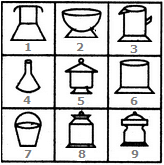Detailed Solution for Grouping Of Images Quiz II, Non Verbal Reasoning - Question 7

5, 8, 9 are objects having both base as well as upper lid.

2, 3, 6 are objects having base but not upper lid.

1, 4, 7 tare objects which have neither a base nor an upper lid attached to them.

Grouping Of Images Quiz II, Non Verbal Reasoning - Question 8
Directions to Solve

In each of the following questions, group the given figures into three classes using each figure only once.

Question -

Group the given figures into three classes using each figure only once.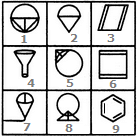Detailed Solution for Grouping Of Images Quiz II, Non Verbal Reasoning - Question 8

3, 6, 9 are geometrical figures containing line segments (the number of these line segments is half the number of sides in the figure) parallel to the sides of the figure.

1, 5, 8 consist of a circle and a triangle intersecting it. The triangle is also divided into two equal parts by a straight line.

2, 4, 7 are all funnel shaped figures.

Grouping Of Images Quiz II, Non Verbal Reasoning - Question 9
Directions to Solve

In each of the following questions, group the given figures into three classes using each figure only once.

Question -

Group the given figures into three classes using each figure only once.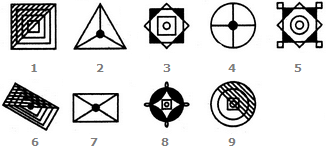Detailed Solution for Grouping Of Images Quiz II, Non Verbal Reasoning - Question 9

1, 6, 9 are figures which are half shaded by slanting lines.

2, 4, 7 are all divided into equal parts (either three or four parts) by straight lines and also have a black circle at the centre.

3, 5, 8 have similar designs and have their four corners shaded black.

Grouping Of Images Quiz II, Non Verbal Reasoning - Question 10
Directions to Solve

In each of the following questions, group the given figures into three classes using each figure only once.

Question -

Group the given figures into three classes using each figure only once.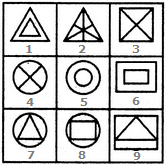Detailed Solution for Grouping Of Images Quiz II, Non Verbal Reasoning - Question 10

1, 5, 6 have two similar elements, one inside the other.

2, 3, 4 contain straight lines each dividing, the figure into two equal parts.

7, 8, 9 have one element placed inside a different element.

## General Aptitude for GATE

84 videos|84 docs|108 tests
Information about Grouping Of Images Quiz II, Non Verbal Reasoning Page
In this test you can find the Exam questions for Grouping Of Images Quiz II, Non Verbal Reasoning solved & explained in the simplest way possible. Besides giving Questions and answers for Grouping Of Images Quiz II, Non Verbal Reasoning, EduRev gives you an ample number of Online tests for practice

## General Aptitude for GATE

84 videos|84 docs|108 tests